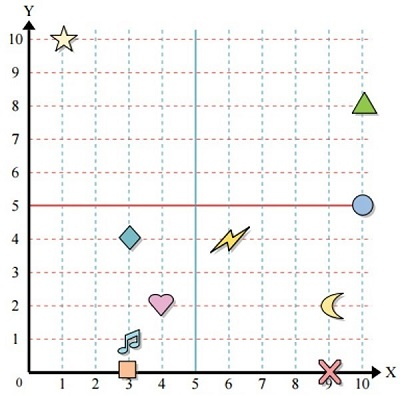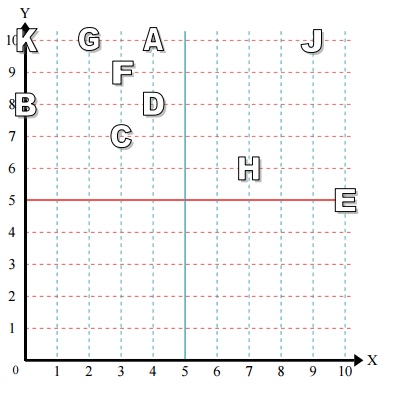A two dimensional coordinate plane is divided by horizontal line and vertical line into four quadrants. The horizontal line is the x-axis and the vertical line is the y axis. The x axis and the y axis intersect at a point called origin.

Every point in the plane is represented by a x coordinate and a y coordinate. Together these coordinates are written in parentheses separated by a comma and are called an ordered pair. The origin has the coordinates (0, 0)

We read the position of a point in a plane or a quadrant in terms of an ordered pair, i.e., x and y coordinate.

In the quadrant 1, both the x coordinate and the y coordinate are positive.

The signs of the ordered pairs in quadrant 1 are (+, +)

We read the number of units the point is to the right of origin along the x axis to find its x coordinate.

We then read the number of units the point is above the origin along the y axis to find its y coordinate.

The x and y coordinates of the given point give its location in terms of an ordered pair. This is how a point in quadrant 1 is read.

Use the grid given below to read the coordinates of the points represented by the shapes the circle, the square, the heart and the triangle.### Solution

Step 1 − The x and y coordinates of the point represented by the circle in the given grid are 10 and 5 respectively. So as an ordered pair, they are (10, 5).

Step 2 − The x and y coordinates of the point represented by the square in the given grid are 3 and 0 respectively. So as an ordered pair, they are (3, 0).

Step 3 − The x and y coordinates of the point represented by the heart in the given grid are 4 and 2 respectively. So as an ordered pair, they are (4, 2).

Step 4 − The x and y coordinates of the point represented by the triangle in the given grid are 10 and 8 respectively. So as an ordered pair, they are (10, 8).

Use the grid given below to read the coordinates of the points represented by the letters, H, A, F and B.### Solution

Step 1 − he x and y coordinates of the point represented by the letter H in the given grid are 7 and 6 respectively. So as an ordered pair, they are (7, 6).

Step 2 − The x and y coordinates of the point represented by the letter A in the given grid are 4 and 10 respectively. So as an ordered pair, they are (4, 10).

Step 3 − The x and y coordinates of the point represented by the letter F in the given grid are 3 and 9 respectively. So as an ordered pair, they are (3, 9).

Step 4 − The x and y coordinates of the point represented by the letter B in the given grid are 0 and 8 respectively. So as an ordered pair, they are (0, 8).